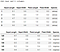# K-nearest neighbors algorithm

K-nearest neighbors (KNN) as the name suggests is the machine learning algorithm to label or predict the value of a data point on the basis of its K-nearest neighbors. Let’s take an example: A person in a college hostel meets a lot of people daily, he has a lot of friends but some of them are his very close friends. Suppose he has five close friends. Out of five, two are very hardworking and studious while the rest of the three are very lazy and they don’t study at all. So according to the KNN algorithm, you will also be classified as lazy because three of the K (5) nearest people around you are lazy. So this was in layman’s terms now let’s come to the machine learning definition of KNN.

KNN is the supervised machine learning algorithm used for classification and regression problems based on the similarity between K nearest neighbors.
Now the question comes into mind, what is the supervised machine learning algorithm?
The supervised machine learning algorithms are those which require both input and output variables for training. In supervised learning, the correct labels or target values should be known initially so that we can supervise how well our model is fitting the data and predicting the target values or class labels. If the model is not predicting target values correctly, we can consider changing the parameters. Unsupervised algorithms are those which require only the input variables to identify the underlying distribution and clustering of the dataset.

## How does it work?

• Euclidean distance is calculated between the new data point and the available data points.
• The K data points having the smallest distances are then selected.
• The mode of the labels of the K data points is taken for the classification problem.
• The mean of the target values of these data points is taken if the problem is regression.

Let’s just use KNN on the iris dataset. Iris dataset contains the features sepal length, sepal width, petal length, and petal width. The labels are the name of the species which are Setosa, Versicolor, and Virginica.

Here is a glimpse of the dataset:Iris dataset

This is a classification problem in which the Species of the given flower needs to be classified on the basis of other features:

• Extract all the columns except Species as X (independent variables) and the Species column as y (dependent variable). And also encode y using label encoders.
• Split the dataset into train and test sets.
• Normalize X_train and X_test using MinMaxScaler.
• Select an appropriate value of K, later on, we will try to find the optimal value of K. Fit the KNN model on X_train and y_train and do the predictions for X_test. Calculate the accuracy score.
• To find the optimal value of K, calculate the accuracy for different values of K. The value of K which gives the maximum accuracy should be chosen. Note that taking K=1 would not be of any help because there are chances that the data point nearest to our test data point belongs to a different class and hence our test data point will be misclassified.
• The plot can be seen here. From the plot, it is quite clear that at K=22 and then at K=25 the maximum accuracy is achieved.

## Why is KNN called a lazy algorithm?

KNN is also known as a lazy algorithm as it doesn’t require any training. In the training phase, it just memorizes the dataset while other algorithms develop discriminative functions and learn model weights from the training data.

KNN does all the hard work in the testing phase. In the testing phase euclidean distance between each of the test data points and all the training data points needs to be computed and then a certain label is assigned to the test data point on the basis of K-nearest training data points. That’s why it is called a lazy algorithm as it does no preparation at the time of training and the complete algorithm is implemented in the testing phase.

## Mathematics behind KNN algorithm:

In KNN we need to find the Euclidean distance between the points. This distance can easily be calculated using the formula:

Where {x1, x2, ………………xn} belongs to X and {y1, y2, ………………yn} belongs to Y . Here X can be taken as a single training sample and Y can be interpreted as a test data point both having n features. There will be a lot of training samples and we need to calculate the distances of our data point from all the training samples.

Other distances like manhattan distance and cosine distance can also be used but euclidean distance works fine.

After calculating distances, the K lowest distances and training data points corresponding to them are selected. The labels or class of these data points are noted down. The class which has the largest probability is then assigned to our test data point. The probability of any class Ci can be calculated as:

Where ni is the number of data points that belong to class Ci given the test data point Y.

Advantages and Applications of KNN:

• KNN is a very simple and easy-to-use algorithm.
• KNN doesn’t require any parameter tuning and expensive training like the other complex algorithms.
• KNN can be used where data needs to be classified on the basis of similarity like in movie recommendation systems.

Disadvantages of KNN:

Although KNN is a very simple algorithm and doesn’t require training it becomes slower when the data size is large. It is because KNN computes the Euclidean distance for each of the test data points from all the training data points hence the time complexity and runtime of the model increases as the size of the data increases.

I like to read and write.

## More from Amansingh

I like to read and write.